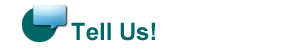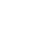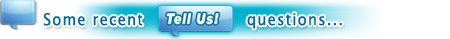\n"); print(" \n"); print(" \n"); print("\n"); print("\n"); print("\n"); print("\n"); \$j++; } ?>Percent of vote2 and (\$stat != 100)) { \$stat = substr(\$stat,0,strpos(\$stat,".")); } } else { \$stat = "0"; } print("
\$j) \$arow \$arow\n"); printf("%.1f", "\$stat "); print(" %\n"); print("\n"); echo ""; print("\n"); print(" \n"); print(" \n"); } } ?>
 \n"); print("\$row